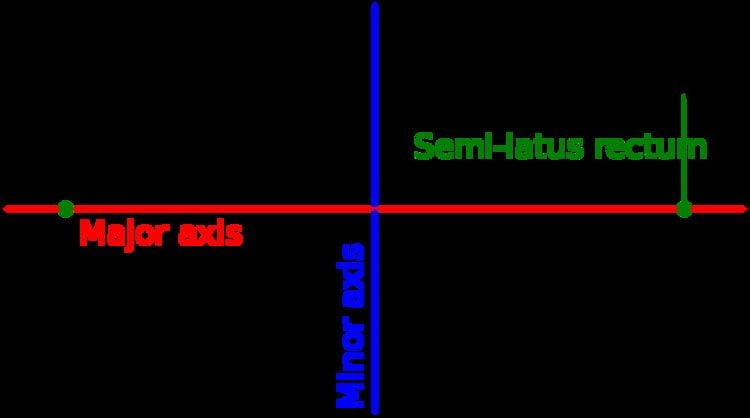# Aspect ratio

Updated onThe aspect ratio of a geometric shape is the ratio of its sizes in different dimensions. For example, the aspect ratio of a rectangle is the ratio of its longer side to its shorter side – the ratio of width to height, when the rectangle is oriented as a "landscape".

## Contents

The aspect ratio is expressed as two numbers separated by a colon (x:y). The values x and y do not represent actual widths and heights but, rather, the relationship between width and height. As an example, 8:5, 16:10 and 1.6:1 are three ways of representing the same aspect ratio.

In objects of more than two dimensions, such as hyperrectangles, the aspect ratio can still be defined as the ratio of the longest side to the shortest side.

## Applications and uses

The term is most commonly used with reference to:

• Graphic / image
• Image aspect ratio
• Display aspect ratio: the aspect ratio for computer displays.
• Paper size
• Standard photographic print sizes
• Motion picture film formats
• Pixel aspect ratio
• Photolithography: the aspect ratio of an etched, or deposited structure is the ratio of the height of its vertical side wall to its width.
• HARMST High Aspect Ratios allow the construction of tall structures without slant
• Tire code
• Tire sizing
• Wing aspect ratio of an aircraft or bird
• Astigmatism of an optical lens
• Nanorod dimensions
• Shape factor (image analysis and microscopy)
• ## Rectangles

For a rectangle, the aspect ratio denotes the ratio of the width to the height of the rectangle. A square has the smallest possible aspect ratio of 1:1.

Examples:

• 4:3 = 1.3: Some (not all) 20th century computer monitors (VGA, XGA, etc.), standard-definition television
• √2:1 = 1.414…: International paper sizes (ISO 216)
• 3:2 = 1.5: 35mm still camera film, iPhone (until iPhone 5) displays
• 16:10 = 1.6 (not shown above): Commonly used widescreen computer displays (WXGA)
• Φ:1 = 1.618…: Golden ratio, close to 16:10
• 5:3 = 1.6: Super 16 mm, a standard film gauge in many European countries
• 16:9 = 1.7: Widescreen TV
• 2:1 = 2: dominoes
• ## Ellipses

For an ellipse, the aspect ratio denotes the ratio of the major axis to the minor axis. An ellipse with an aspect ratio of 1:1 is a circle.

## Aspect ratios of general shapes

In geometry, there are several alternative definitions to aspect ratios of general compact sets in a d-dimensional space:

• The Diameter-Width Aspect Ratio (DWAR) of a compact set is the ratio of its diameter to its width. A circle has the minimal DWAR which is 1. A square has a DWAR of sqrt(2).
• The Cube-Volume Aspect Ratio (CVAR) of a compact set is the d-th root of the ratio of the d-volume of the smallest enclosing axes-parallel d-cube, to the set's own d-volume. A square has the minimal CVAR which is 1. A circle has a CVAR of sqrt(2). An axis-parallel rectangle of width W and height H, where W>H, has a CVAR of sqrt(W^2/WH) = sqrt(W/H).
• If the dimension d is fixed, then all reasonable definitions of aspect ratio are equivalent to within constant factors.

## Notations

Aspect ratios are mathematically expressed as x:y (pronounced "x-to-y").

Cinematographic aspect ratios are usually denoted as a (rounded) decimal multiple of width vs unit height, while photographic and videographic aspect ratios are usually defined and denoted by whole number ratios of width to height. In digital images there is a subtle distinction between the Display Aspect Ratio (the image as displayed) and the Storage Aspect Ratio (the ratio of pixel dimensions); see Distinctions.

Topics